# surfnorm

Surface normals

## Syntax

``surfnorm(X,Y,Z)``
``surfnorm(Z)``
``surfnorm(ax,___)``
``surfnorm(___,Name,Value)``
``[Nx,Ny,Nz] = surfnorm(___)``

## Description

example

````surfnorm(X,Y,Z)` creates a three-dimensional surface plot and displays its surface normals. A surface normal is the imaginary line perpendicular to a flat surface, or perpendicular to the tangent plane at a point on a non-flat surface.The function plots the values in matrix `Z` as heights above a grid in the x-y plane defined by `X` and `Y`. The color of the surface varies according to the heights specified by `Z`. The matrices `X`, `Y`, and `Z` must be the same size.```
````surfnorm(Z)` creates a surface with normals and uses the column and row indices of the elements in `Z` as the x and y-coordinates, respectively.```
````surfnorm(ax,___)` plots into the axes specified by `ax` instead of the current axes. Specify the axes as the first input argument.```

example

````surfnorm(___,Name,Value)` specifies surface properties using one or more name-value pair arguments. For example, `'FaceAlpha',0.5` creates a semitransparent surface.```

example

````[Nx,Ny,Nz] = surfnorm(___)` returns the x, y, and z components of the three-dimensional surface normals for the surface without plotting anything.```

## Examples

collapse all

Create a cone. Then plot the data as a surface and display the surface normals. The surface uses `Z` for both height and color.

```[X,Y,Z] = cylinder(1:10); surfnorm(X,Y,Z)```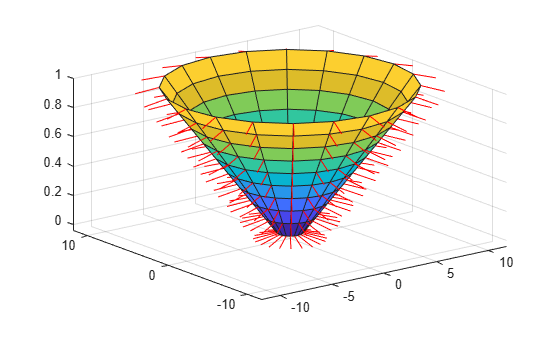Create a surface with no edges by specifying the `EdgeColor` name-value pair with `'none'` as the value.

```[X,Y,Z] = cylinder(1:10); surfnorm(X,Y,Z,'EdgeColor','none')```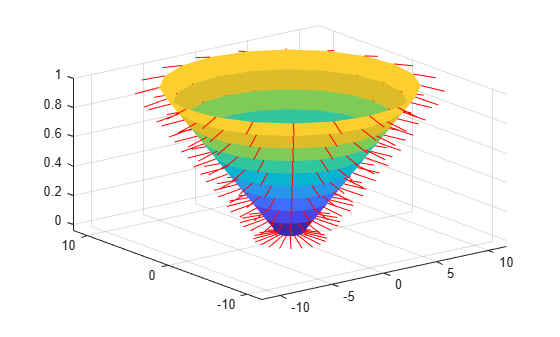Use the surface normals of a curved surface to light a flat surface.

First, display a flat surface.

`surf(ones(49),'EdgeColor','none');`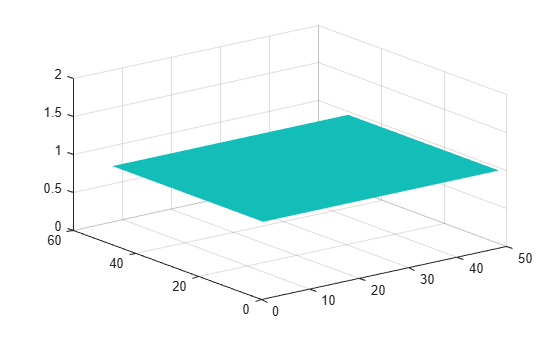Display the curved surface to use as a lighting source.

`surf(peaks);`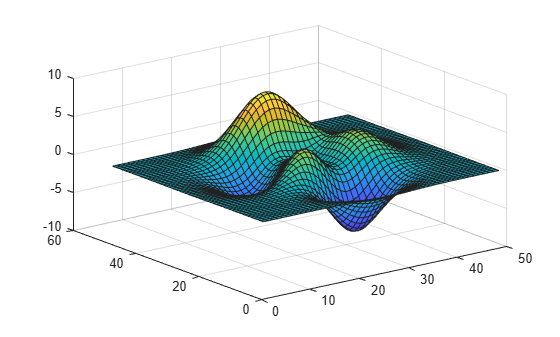Now, draw the flat surface again, this time with lighting from the curved surface. To do this, first calculate the surface normals of the curved surface.

`[nx, ny, nz] = surfnorm(peaks);`

Combine the x, y, and z surface normal components into a single 49-by-49-by-3 array.

`b = reshape([nx ny nz], 49,49,3);`

Create a flat surface again, this time supplying this array as a value for the `VertexNormals` property. MATLAB® uses the `VertexNormals` property to calculate the surface lighting. Set the lighting algorithm to `gouraud` and add a light using `camlight`.

```surf(ones(49),'VertexNormals',b,'EdgeColor','none'); lighting gouraud camlight```## Input Arguments

collapse all

x-coordinates, specified as a matrix the same size as `Y` and `Z`.

You can use the `meshgrid` function to create the `X` and `Y` matrices.

The `XData` property of the `Surface` object stores the x-coordinates.

Example: `X = [1 2 3; 1 2 3; 1 2 3]`

Example: `[X,Y] = meshgrid(-5:0.5:5)`

Data Types: `single` | `double` | `int8` | `int16` | `int32` | `int64` | `uint8` | `uint16` | `uint32` | `uint64`

y-coordinates, specified as a matrix the same size as `X` and `Z`.

You can use the `meshgrid` function to create the `X` and `Y` matrices.

The `YData` property of the surface object stores the y-coordinates.

Example: `Y = [1 1 1; 2 2 2; 3 3 3]`

Example: `[X,Y] = meshgrid(-5:0.5:5)`

Data Types: `single` | `double` | `int8` | `int16` | `int32` | `int64` | `uint8` | `uint16` | `uint32` | `uint64`

z-coordinates, specified as a matrix. `Z` must have at least three rows and three columns. `Z` also sets the surface colors.

The `ZData` property of the surface object stores the z-coordinates.

Example: `Z = [1 2 3; 4 5 6; 7 8 9]`

Data Types: `single` | `double` | `int8` | `int16` | `int32` | `int64` | `uint8` | `uint16` | `uint32` | `uint64`

Axes to plot in, specified as an `axes` object. If you do not specify the axes, then `surfnorm` plots into the current axes.

### Name-Value Arguments

Specify optional pairs of arguments as `Name1=Value1,...,NameN=ValueN`, where `Name` is the argument name and `Value` is the corresponding value. Name-value arguments must appear after other arguments, but the order of the pairs does not matter.

Before R2021a, use commas to separate each name and value, and enclose `Name` in quotes.

Example: `surfnorm(X,Y,Z,'FaceAlpha',0.5,'EdgeColor','none')` creates a semitransparent surface with no edges drawn.

Note

The properties listed here are only a subset. For a full list, see Surface Properties.

Edge line color, specified as one of the values listed here. The default color of `[0 0 0]` corresponds to black edges.

ValueDescription
`'none'`Do not draw the edges.
`'flat'`

Use a different color for each edge based on the values in the `CData` property. First you must specify the `CData` property as a matrix the same size as `ZData`. The color value at the first vertex of each face (in the positive x and y directions) determines the color for the adjacent edges. You cannot use this value when the `EdgeAlpha` property is set to `'interp'`.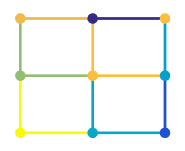`'interp'`

Use interpolated coloring for each edge based on the values in the `CData` property. First you must specify the `CData` property as a matrix the same size as `ZData`. The color varies across each edge by linearly interpolating the color values at the vertices. You cannot use this value when the `EdgeAlpha` property is set to `'flat'`.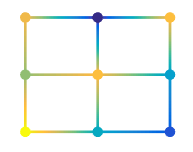RGB triplet, hexadecimal color code, or color name

Use the specified color for all the edges. This option does not use the color values in the `CData` property.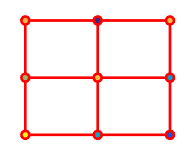RGB triplets and hexadecimal color codes are useful for specifying custom colors.

• An RGB triplet is a three-element row vector whose elements specify the intensities of the red, green, and blue components of the color. The intensities must be in the range `[0,1]`; for example,``` [0.4 0.6 0.7]```.

• A hexadecimal color code is a character vector or a string scalar that starts with a hash symbol (`#`) followed by three or six hexadecimal digits, which can range from `0` to `F`. The values are not case sensitive. Thus, the color codes `"#FF8800"`, `"#ff8800"`, `"#F80"`, and `"#f80"` are equivalent.

Alternatively, you can specify some common colors by name. This table lists the named color options, the equivalent RGB triplets, and hexadecimal color codes.

Color NameShort NameRGB TripletHexadecimal Color CodeAppearance
`"red"``"r"``[1 0 0]``"#FF0000"``"green"``"g"``[0 1 0]``"#00FF00"``"blue"``"b"``[0 0 1]``"#0000FF"``"cyan"` `"c"``[0 1 1]``"#00FFFF"``"magenta"``"m"``[1 0 1]``"#FF00FF"``"yellow"``"y"``[1 1 0]``"#FFFF00"``"black"``"k"``[0 0 0]``"#000000"``"white"``"w"``[1 1 1]``"#FFFFFF"`Here are the RGB triplets and hexadecimal color codes for the default colors MATLAB® uses in many types of plots.

`[0 0.4470 0.7410]``"#0072BD"``[0.8500 0.3250 0.0980]``"#D95319"``[0.9290 0.6940 0.1250]``"#EDB120"``[0.4940 0.1840 0.5560]``"#7E2F8E"``[0.4660 0.6740 0.1880]``"#77AC30"``[0.3010 0.7450 0.9330]``"#4DBEEE"``[0.6350 0.0780 0.1840]``"#A2142F"`Line style, specified as one of the options listed in this table.

Line StyleDescriptionResulting Line
`"-"`Solid line`"--"`Dashed line`":"`Dotted line`"-."`Dash-dotted line`"none"`No lineNo line

Face color, specified as one of the values in this table.

ValueDescription
`'flat'`

Use a different color for each face based on the values in the `CData` property. First you must specify the `CData` property as a matrix the same size as `ZData`. The color value at the first vertex of each face (in the positive x and y directions) determines the color for the entire face. You cannot use this value when the `FaceAlpha` property is set to `'interp'`.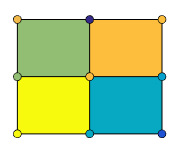`'interp'`

Use interpolated coloring for each face based on the values in the `CData` property. First you must specify the `CData` property as a matrix the same size as `ZData`. The color varies across each face by interpolating the color values at the vertices. You cannot use this value when the `FaceAlpha` property is set to `'flat'`.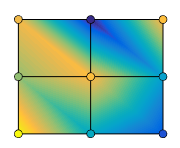RGB triplet, hexadecimal color code, or color name

Use the specified color for all the faces. This option does not use the color values in the `CData` property.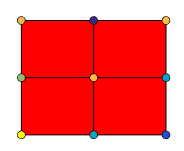`'texturemap'`Transform the color data in `CData` so that it conforms to the surface.
`'none'`Do not draw the faces.

RGB triplets and hexadecimal color codes are useful for specifying custom colors.

• An RGB triplet is a three-element row vector whose elements specify the intensities of the red, green, and blue components of the color. The intensities must be in the range `[0,1]`; for example,``` [0.4 0.6 0.7]```.

• A hexadecimal color code is a character vector or a string scalar that starts with a hash symbol (`#`) followed by three or six hexadecimal digits, which can range from `0` to `F`. The values are not case sensitive. Thus, the color codes `"#FF8800"`, `"#ff8800"`, `"#F80"`, and `"#f80"` are equivalent.

Alternatively, you can specify some common colors by name. This table lists the named color options, the equivalent RGB triplets, and hexadecimal color codes.

Color NameShort NameRGB TripletHexadecimal Color CodeAppearance
`"red"``"r"``[1 0 0]``"#FF0000"``"green"``"g"``[0 1 0]``"#00FF00"``"blue"``"b"``[0 0 1]``"#0000FF"``"cyan"` `"c"``[0 1 1]``"#00FFFF"``"magenta"``"m"``[1 0 1]``"#FF00FF"``"yellow"``"y"``[1 1 0]``"#FFFF00"``"black"``"k"``[0 0 0]``"#000000"``"white"``"w"``[1 1 1]``"#FFFFFF"`Here are the RGB triplets and hexadecimal color codes for the default colors MATLAB uses in many types of plots.

`[0 0.4470 0.7410]``"#0072BD"``[0.8500 0.3250 0.0980]``"#D95319"``[0.9290 0.6940 0.1250]``"#EDB120"``[0.4940 0.1840 0.5560]``"#7E2F8E"``[0.4660 0.6740 0.1880]``"#77AC30"``[0.3010 0.7450 0.9330]``"#4DBEEE"``[0.6350 0.0780 0.1840]``"#A2142F"`Face transparency, specified as one of these values:

• Scalar in range `[0,1]` — Use uniform transparency across all the faces. A value of `1` is fully opaque and `0` is completely transparent. Values between `0` and `1` are semitransparent. This option does not use the transparency values in the `AlphaData` property.

• `'flat'` — Use a different transparency for each face based on the values in the `AlphaData` property. The transparency value at the first vertex determines the transparency for the entire face. First you must specify the `AlphaData` property as a matrix the same size as the `ZData` property. The `FaceColor` property also must be set to `'flat'`.

• `'interp'` — Use interpolated transparency for each face based on the values in `AlphaData` property. The transparency varies across each face by interpolating the values at the vertices. First you must specify the `AlphaData` property as a matrix the same size as the `ZData` property. The `FaceColor` property also must be set to `'interp'`.

• `'texturemap'` — Transform the data in `AlphaData` so that it conforms to the surface.

Effect of light objects on faces, specified as one of these values:

• `'flat'` — Apply light uniformly across each face. Use this value to view faceted objects.

• `'gouraud'` — Vary the light across the faces. Calculate the light at the vertices and then linearly interpolate the light across the faces. Use this value to view curved surfaces.

• `'none'` — Do not apply light from light objects to the faces.

To add a light object to the axes, use the `light` function.

Note

The `'phong'` value has been removed. Use `'gouraud'` instead.

## Output Arguments

collapse all

Surface normal x-component, returned as a matrix. For more information about how the surface normals are computed, see Algorithms.

Surface normal y-component, returned as a matrix. For more information about how the surface normals are computed, see Algorithms.

Surface normal z-component, returned as a matrix. For more information about how the surface normals are computed, see Algorithms.

## Tips

• To reverse the direction of the normals, call `surfnorm` with transposed arguments:

```surfnorm(X',Y',Z') ```

• To show the direction of the normals on a surface, use the `surfnorm` function to calculate the surface normals and then the `quiver3` function to display them.

```[Nx,Ny,Nz] = surfnorm(X,Y,Z); quiver3(X,Y,Z,Nx,Ny,Nz) ```

• The surface normals represent conditions at vertices and are not normalized. Normals for surface elements that face away from the viewer are not visible.

## Algorithms

`surfnorm` uses bicubic interpolation in the x, y, and z directions to calculate the surface normals of the data. To allow for interpolation at the boundaries, the function uses quadratic extrapolation to expand the data. After performing the bicubic fit of the data, diagonal vectors are computed and crossed to form the normal at each vertex.

## Version History

Introduced before R2006a